The role of a catalyst is to change

1.  Gibbs energy of the reaction

2.  Enthalpy of reaction

3.  The activation energy of the reaction

4.  Equilibrium constant

Subtopic:  Catalyst |
Please attempt this question first.
Please attempt this question first.
Please attempt this question first.
Launched MCQ Practice Books

Prefer Books for Question Practice? Get NEETprep's Unique MCQ Books with Online Video/Text Solutions via Telegram Bot

In the presence of a catalyst, the heat evolved or absorbed during the reaction

1.  Increases

2.  Decreases

3.  Remains unchanged

4.  May increase or decrease

Subtopic:  Catalyst |
Please attempt this question first.
Please attempt this question first.
Please attempt this question first.
Launched MCQ Practice Books

Prefer Books for Question Practice? Get NEETprep's Unique MCQ Books with Online Video/Text Solutions via Telegram Bot

The activation energy of a chemical reaction can be calculated by

1.  Determining the rate constant at standard temperature.

2.  Determining the rate constant at two temperatures.

3.  Determining probability of collision.

4.  Using the catalyst.

Subtopic:  Arrhenius Equation |
Please attempt this question first.
Please attempt this question first.
Please attempt this question first.
Launched MCQ Practice Books

Prefer Books for Question Practice? Get NEETprep's Unique MCQ Books with Online Video/Text Solutions via Telegram Bot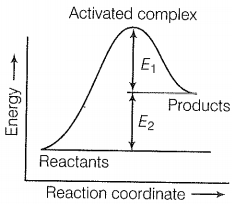The correct statement among the following based on the above mentioned graph is:

1. The activation energy of the forward reaction is and the product is less stable than reactant.

2. The activation energy of the forward reaction is and the product is more stable than the reactant.

3. The activation energy of both forward and backward reaction is and reactant is more stable than the product.

4.  The activation energy of the backward reaction is E1 and the product is more stable than reactant.

Subtopic:  Arrhenius Equation |
Please attempt this question first.
Please attempt this question first.
Please attempt this question first.
Launched MCQ Practice Books

Prefer Books for Question Practice? Get NEETprep's Unique MCQ Books with Online Video/Text Solutions via Telegram Bot

Consider the first-order gas-phase decomposition reaction given below.

$A\left(g\right)\to B\left(g\right)+C\left(g\right)$

The initial pressure of the system before the decomposition of A was ${\mathrm{P}}_{\mathrm{i}}$. After the lapse of time t, the total pressure of the system increased by X units and became ${\mathrm{P}}_{\mathrm{t}}$. The rate constant k for the reaction is:

1. $\mathrm{k}=\frac{2.303}{\mathrm{t}}\mathrm{log}\frac{{P}_{\mathrm{i}}}{{P}_{\mathrm{i}}-\mathrm{x}}$

2. $\mathrm{k}=\frac{2.303}{\mathrm{t}}\mathrm{log}\frac{{P}_{\mathrm{i}}}{2{P}_{\mathrm{i}}-{P}_{\mathrm{t}}}$

3. $\mathrm{k}=\frac{2.303}{\mathrm{t}}\mathrm{log}\frac{{P}_{\mathrm{i}}}{2{P}_{\mathrm{i}}+{P}_{\mathrm{t}}}$

4. $\mathrm{k}=\frac{2.303}{\mathrm{t}}\mathrm{log}\frac{{P}_{\mathrm{i}}}{{P}_{\mathrm{i}}+\mathrm{x}}$

Subtopic:  First Order Reaction Kinetics |
Please attempt this question first.
Please attempt this question first.
Please attempt this question first.
Launched MCQ Practice Books

Prefer Books for Question Practice? Get NEETprep's Unique MCQ Books with Online Video/Text Solutions via Telegram Bot

Which of the following graphs show the relation between ln k and 1/T?

1.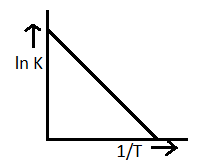2.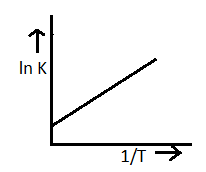3.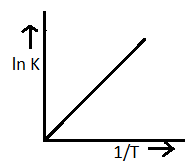4.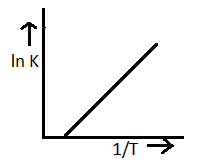Subtopic:  Arrhenius Equation |
Please attempt this question first.
Please attempt this question first.
Please attempt this question first.
Launched MCQ Practice Books

Prefer Books for Question Practice? Get NEETprep's Unique MCQ Books with Online Video/Text Solutions via Telegram Bot

Consider the Arrhenius equation given below and choose the correct option:

$k=A{e}^{-\frac{{E}_{a}}{RT}}$

1. Rate constant increases exponentially with increasing activation energy and decreasing temperature.

2. Rate constant decreases exponentially with increasing activation energy and decreasing temperature.

3. Rate constant increases exponentially with decreasing activation energy and decreasing temperature.

4. Rate constant increases exponentially with decreasing activation energy and increasing temperature.

Subtopic:  Arrhenius Equation |
Please attempt this question first.
Please attempt this question first.
Please attempt this question first.
Launched MCQ Practice Books

Prefer Books for Question Practice? Get NEETprep's Unique MCQ Books with Online Video/Text Solutions via Telegram Bot

A graph of volume of hydrogen released vs time for the reaction between zinc and dil. HCl is given in the graph below.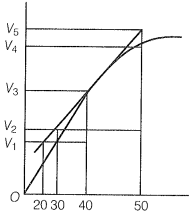The correct statement among the following based on the graph given above is:

Subtopic:  Definition, Rate Constant, Rate Law |
Please attempt this question first.
Please attempt this question first.
Please attempt this question first.
Launched MCQ Practice Books

Prefer Books for Question Practice? Get NEETprep's Unique MCQ Books with Online Video/Text Solutions via Telegram Bot

Which of the following statements is not correct about the order of a reaction?

1. The order of a reaction can be a fractional number

2. Order of a reaction is experimentally determined quantity

3. The order of a reaction is always equal to the sum of the stoichiometric coefficients of reactants in the balanced chemical equation for a reaction

4. The order of a reaction is the sum of the powers of the molar concentration of the reactants in the rate law expression

Subtopic:  Order,Molecularity and Mechanism |
Please attempt this question first.
Please attempt this question first.
Please attempt this question first.
Launched MCQ Practice Books

Prefer Books for Question Practice? Get NEETprep's Unique MCQ Books with Online Video/Text Solutions via Telegram Bot

Consider the graph given in the figure. Which of the following options does not show an instantaneous rate of reaction in the 40s?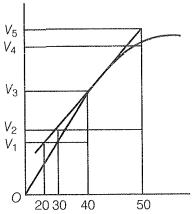Subtopic:  Definition, Rate Constant, Rate Law |
Please attempt this question first.
Please attempt this question first.
Please attempt this question first.
Launched MCQ Practice Books

Prefer Books for Question Practice? Get NEETprep's Unique MCQ Books with Online Video/Text Solutions via Telegram Bot